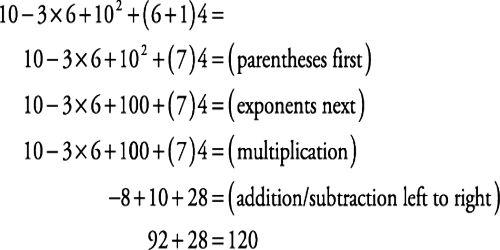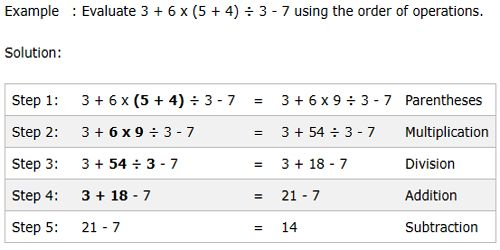Calculations using the Order of Operations

The orders of operations are rules that govern which mathematical operations are done first.

For example, in mathematics and most computer languages, multiplication is granted a higher precedence than addition, and it has been this way since the introduction of modern algebraic notation. Thus, the expression 2 + 3 × 4 is interpreted to have the value 2 + (3 × 4) = 14, not (2 + 3) × 4 = 20. With the introduction of exponents in the 16th and 17th centuries, they were given precedence over both addition and multiplication and could be placed only as a superscript to the right of their base. Thus 3 + 52 = 28 and 3 × 52 = 75.

• Do operations in parentheses and other grouping symbols first. If there are grouping symbols within other grouping symbols do the innermost first.
• Do multiplication and division operations from left to right.
• Do addition and subtraction operations from left to right.Example: 2 + 3 * (4 + (6 * 3 – 8)) * 2

2 + 3 * (4 + (18 – 8)) * 2

2 + 3 * (4 + 10) * 2

2 + 3 * 14 * 2

2 + 42 * 2

2 + 84 = 86

A common technique for remembering the order of operations is the abbreviation (or, more properly, the “acronym”) “PEMDAS”, which is turned into the mnemonic phrase “Please Excuse My Dear Aunt Sally”. This phrase stands for, and helps one remember the order of, “Parentheses, Exponents, Multiplication and Division, and Addition and Subtraction”. This listing tells you the ranks of the operations: Parentheses outrank exponents, which outrank multiplication and division (but multiplication and division are at the same rank), and multiplication and division outrank addition and subtraction (which are together on the bottom rank). In other words, the precedence is:

• Parentheses (simplify inside ’em)
• Exponents
• Multiplication and Division (from left to right)
• Addition and Subtraction (from left to right)

When you have a bunch of operations of the same rank, you just operate from left to right. For instance, 15 ÷ 3 × 4 is not 15 ÷ (3 × 4) = 15 ÷ 12, but is rather (15 ÷ 3) × 4 = 5 × 4, because, going from left to right, you get to the division sign first.Information Source: